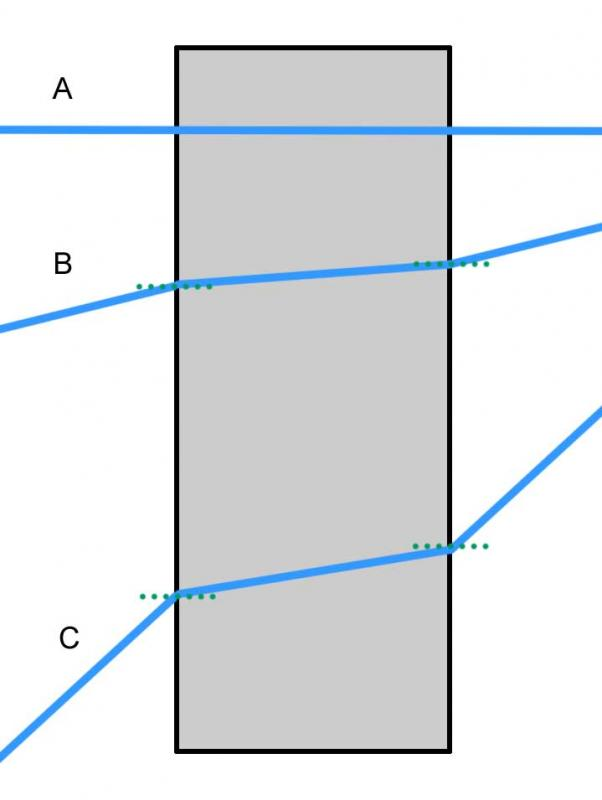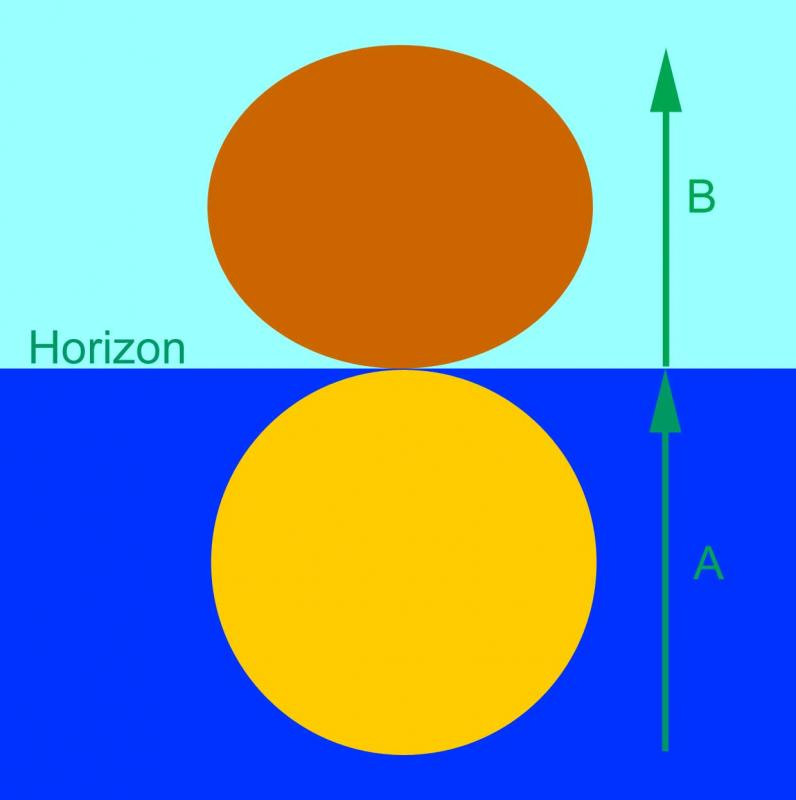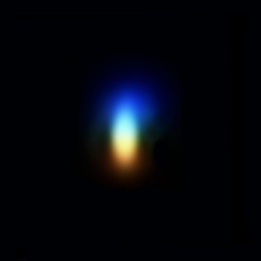## Atmospheric Refraction

This short tutorial looks at the some of the effects that the refraction (bending) of light in the atmosphere has on our view of the sky. These effects include shifting the apparent positions of objects in the heavens, increasing the duration of daylight by moving the times of sunrise and sunset and distorting the apparent shape of the sun and moon when close to the horizon. Lastly the tutorial touches on the way refraction spreads out light into its constituent colours and how this affects the image in a telescope particularly when using long focal lengths.

##### What is refraction?Figure 1. Rays of visible light entering and leaving a glass block at varying angles of incidence.When light travels from an area of one density to another it changes speed. For example visible light passing from air into glass is slowed down. Conversely, when moving from a higher density substance to a lower one, such as light leaving a glass lens and re-entering the air, it speeds up. This change in speed causes the path of the light to be bent or refracted.

The amount of bending is governed by the refractive indices of both substances and what is known as Snell’s law.

The key points are:
1. Light entering or leaving a new medium perpendicular to its surface passes straight though without any deviation (Figure 1 – ray A).
2. At other angles the ray will be refracted and the greater the angle of incidence the greater the amount of refraction (Figure 1 – rays B & C). Notice how the light is bent towards the normal (green lines) on entering the glass and away from it on leaving. This is due to the glass having a higher refractive index than the surroundings and the resultant change in the light’s speed.
3. The refractive index and hence amount of refraction is slightly different for different wavelengths (colours) of light and consequently white light can be dispersed into its constituent colours to form a spectrum.
##### Refraction of light in the atmosphere
Light entering a uniform medium such as glass is the simplest case. However the atmosphere is much more complex. As light moves from the vacuum of outer space into the atmosphere it encounters increasing atmospheric density and with it a changing refractive index. Consequently, as light passes through the atmosphere, the amount of refraction increases causing the light to take on a “curved” path rather than a straight one as is the case with a uniform medium such as glass.Figure 2. An observer at ‘O’ experiences different amounts of refraction depending on an object’s altitude. Star B appears at B’ and C at C’. Not to scale!The schematic in figure 2 shows rays of light from stars at different altitudes above the horizon passing through the atmosphere. The structure of the atmosphere has been very much simplified and the amount of refraction greatly exaggerated. Notice in particular the following:

1. The light from star A, directly overhead, is not refracted and its light reaches the observer without any deviation to its path.
2. The lower the altitude of a star the greater the length of air it has to pass through.
3. The lower the altitude of a star the more obliquely its light strikes the atmosphere.

These last two points combine to cause the amount of refraction to increase rapidly as an object approaches the horizon. As a result star B appears at position B’ and star C is lifted to C’.

##### How much is light refracted?
Over the years a number of different formulae have been developed to answer this question. The underlying problem is achieving a suitable level of accuracy given the complex nature of the Earth’s atmosphere.

As noted above, the amount of refraction is not only dependent on an object’s altitude but also on the density of the atmosphere which in turn is influenced by both temperature and pressure.

A “quick and dirty” formula assuming ‘standard’ temperatures and pressures is:

R = 57 / tan (a)

Where:  R = the amount of refraction in arc seconds.
a = the true altitude of the object.

This is fine as long as temperature and pressure do not stray too far from the standard values but even so it fails completely at an altitude of less than about 20 degrees.

There are a number of more complex equations that give better approximations for example one by Sæmundsson:

R = 1.02*cot {a + [10.3 / (a + 5.11)]} *P /101 * {283 / (273 + T)}

Where:  R = the amount of refraction in arc minutes.
a = the object’s true altitude in degrees.
P = atmospheric pressure in kPa.
T = temperature in Celsius.

An online version of this equation and others is available here (this uses mbar rather than kPa; 1kPa  = 10mbar, it also has the option of using mm of Mercury as often found on barometers).

The following table shows typical refraction in arc minutes and seconds for a variety of altitudes above the horizon at a temperature of 10oC and pressure of 101kPa.
 Altitude Refraction Altitude Refraction 90o 0’ 0” 15o 3’ 41” 80o 0’ 11” 10o 5’ 25” 70o 0’ 22” 5o 9’ 40” 60o 0’ 35” 2o 16’ 56” 50o 0’ 51” 1o 21’ 45” 40o 1’ 12” 0o 30’ 25’ 0” 30o 1’ 45” 0o 28’ 59” 20o 2’ 45” -0o 30’ 33’ 41”

Notice how rapidly the amount of refraction increases as the horizon is approached. Be aware that while this formula can give good results, local atmospheric conditions can at times produce noticeably different refraction particularly at very low altitudes.
##### On the horizonFigure 3. Although the Sun at S is actually below the horizon, refraction lifts it just enough so that it appears to be on the point of setting (S’).Let’s consider the setting Sun. As it approaches the horizon the amount of refraction is around 0.5o, which is roughly the Sun’s apparent diameter. When the Sun appears to be sitting on the horizon, just rising or setting, it is really below the geometric horizon and is only lifted into visibility by atmospheric refraction. Figure 3 shows this situation. If we could miraculously make the Earth’s atmosphere vanish at the apparent moment of sunset or sunrise then the Sun would actually disappear from view as it is really already below the horizon. Obviously this uplift applies to any astronomical object such as stars, planets and the Moon, not just the Sun.

The effect of all this is to lengthen the time an object is above the horizon. In particular, the day becomes longer with the Sun appearing to rise earlier and set later than it would in the absence of an atmosphere.Figure 4. With the Sun just below the horizon, its lower limb is refracted through ‘A’ to rest on the horizon while its upper limb is refracted through the smaller distance ‘B’ to give an oval Sun.Consider the last two entries in the refraction table above. These show the position when Sun seems on to be on the horizon, but is actually below it. Because the altitudes differ by 0.5o which is about the Sun’s apparent diameter, the lower limb of the Sun is lifted by roughly 34’ and the upper by ‘only’ 29’. With the refraction at the Sun’s lower limb being greater than that at the upper, the lower limb is uplifted by about 16% more than the upper and the normally round solar disk is flattened into an oval shape. This is very much the ideal situation; in reality discrete layers in the atmosphere can have different effects often causing a jagged edge to the solar limb. Also local conditions can cause the amount of refraction to vary significantly at such low altitudes. The same effect can be seen with the Moon.
##### Tracking across the sky

Consider the case of a star moving across the sky. This is usually shown as a smooth curve with the star rising in the eastern part of the sky, reaching its highest point on the meridian and then sinking towards the western horizon. This is a reasonable approximation to reality and is perfectly correct in the absence of the atmosphere. Taking refraction into account we find that any star, except at the zenith, is higher than it would be and the difference varies with its altitude. Consequently its path across the sky is more complex.

Does this matter? The answer is yes if you have a telescope drive that is attempting to track the stars. The continually changing amount of refraction means that the telescope will not keep a star perfectly centred in the field of view. For purely visual observing this is something that is easy to cope with but for photography it does present a challenge. With an equatorial mount that needs to be aligned on the celestial pole the best thing to do is to align on the refracted pole not the geometric one. Most methods that use star alignment will do this automatically but if you are using a mechanical method such as an inclinometer you could make an adjustment for refraction particularly at low latitudes.

##### Atmospheric DispersionFigure 5. An image of Polaris at high magnification showing atmospheric dispersion. Image courtesy of Martin Lewis.At the beginning of this tutorial we noted that the amount light is refracted varies slightly with its wavelength (colour). This can cause a star’s image to be spread out into a very small spectrum with red at the top and blue at the bottom. This effect, only really visible in a telescope, increases as an object’s altitude decreases. Figure 5 shows the effect on Polaris at an altitude of 52o. The image here is greatly enlarged and because it is taken through an inverting telescope the colours are reversed from those above with red at the bottom and blue at the top.

The situation with planets is more difficult particularly at the long focal lengths and large image scales used in imaging. Here the single image is comprised of multiple images of different colours all slightly out of alignment with one another blurring the detail. For a more detailed discussion of atmospheric dispersion and ways of counteracting it Martin Lewis has written an in depth article available here and there is also one by Damian Peach here.

##### Conclusion

Even though atmospheric refraction can often go unnoticed the effects it causes can be quite significant. From affecting the way a telescope tracks to smearing planetary images and even extending daylight, its effects can be surprising.